Next: Vector Line Integrals Up: Vector Algebra and Vector Previous: Vector Calculus

Line Integrals

Consider a two-dimensional function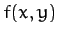which is defined for alland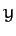. What is meant by the integral of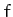along a given curve joining the pointsand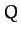in the-plane? Well, we first draw outas a function of lengthalong the path--see Figure A.107. The integral is then simply given by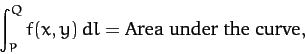(1326)

where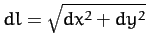.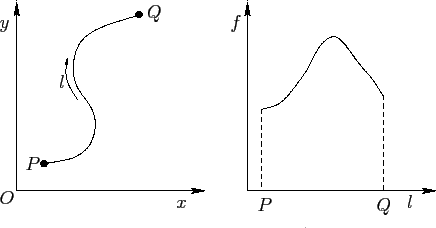As an example of this, consider the integral of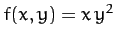betweenandalong the two routes indicated in Figure A.108. Along route 1 we have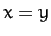, so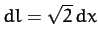. Thus,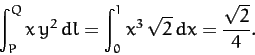(1327)

The integration along route 2 gives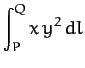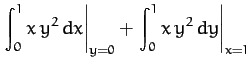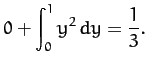(1328)

Note that the integral depends on the route taken between the initial and final points.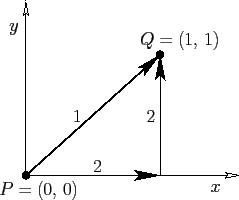The most common type of line integral is that in which the contributions from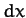and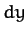are evaluated separately, rather that through the path length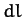: i.e.,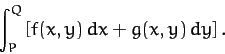(1329)

As an example of this, consider the integral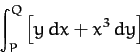(1330)

along the two routes indicated in Figure A.109. Along route 1 we have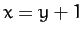and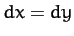, so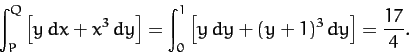(1331)

Along route 2,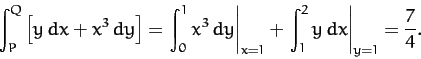(1332)

Again, the integral depends on the path of integration.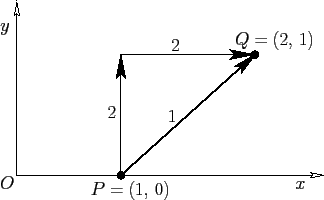Suppose that we have a line integral which does not depend on the path of integration. It follows that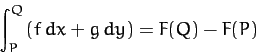(1333)

for some function. Given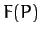for one pointin the-plane, then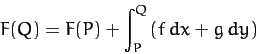(1334)

defines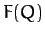for all other points in the plane. We can then draw a contour map of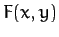. The line integral between pointsandis simply the change in height in the contour map between these two points: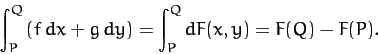(1335)

Thus,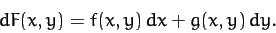(1336)

For instance, if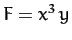then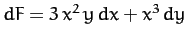and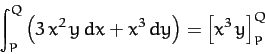(1337)

is independent of the path of integration.

It is clear that there are two distinct types of line integral. Those which depend only on their endpoints and not on the path of integration, and those which depend both on their endpoints and the integration path. Later on, we shall learn how to distinguish between these two types (see Section A.15).Next: Vector Line Integrals Up: Vector Algebra and Vector Previous: Vector Calculus
Richard Fitzpatrick 2011-03-31